# Cervical-Occipital Assembly Movements of the Upper Cervical Assembly

 Page 6/14 Date 23.04.2018 Size 1.62 Mb. #45829

## Framed Vectors

The model used in this paper is based on the manipulation of framed vectors, associated with the vertebrae, by rotations about a set of axes of rotation. A framed vector is a collection of vectors that characterize the location, extension, and orientation of a moving rigid body. The framed vector for a vertebra might have 1.) a location vector, from the origin of the universal coordinate system to the location of some feature of the vertebra, 2.) an extension vector or vectors, between two features of the vertebra that are relevant to its size and shape, and 3). A frame of reference, for the vertebra. The frame of reference is a set of three vectors that lie in particular directions relative to the vertebra. For instance, one might choose a sagittal vector that extends perpendicular to the anterior surface of the vertebra, a vertical or longitudinal vector, that extends perpendicular to the superior surface of the vertebra, and a transverse vector that extends perpendicular to the other two.

It is sufficient that the three vectors in the frame of reference be independent, that is, none of the vectors can be expressed as a linear combination of the other two, therefore they are not coplanar. However, the frame of reference tends to be most useful if it is composed of three orthogonal unit vectors. It also tends to most convenient for understanding the consequences of movements if one chooses them to correspond to anatomically interesting features of the vertebra.

Note that there are two different possible orderings for the frame of reference. If one places one’s right thumb so that it points in the direction of the positive sagittal vector then, if rotation of the positive transverse axis through 90° in the direction that the fingers curl will bring it into alignment with the positive vertical axis, then the frame of reference is said to be right-handed. If using the left hand produces the alignment, then the frame of reference is said to be left-handed. One may choose to use either system, depending on the situation, but once chosen all the results are contingent upon that choice. You must stay in the same system when interpreting the results.

This set of five vectors is the standard form for a framed vector in that it codifies the necessary information for understanding movements of a rigid body. However, it is often convenient to add one or more additional vectors to encapsulate all the features that one wishes to monitor. For instance, one might have several extension vectors to follow how various points on the vertebra, such as the facets and the spine, move in space. It is possible that one would use an entire array of vectors that describe the shape of the vertebra. Alternatively, one might use multiple location vectors to different features of the vertebra. Generally, one would not use multiple frames of reference, but it easy to envision how doing so might give useful information.

The location vector, extension vector, and frame of reference are modified in different ways by movement, therefore it is necessary to combine them in different ways with the quaternions that represent rotations.

## Structural elements

We normally start from the support element and work our way through the joint elements. We assume that there is a neutral state for the system and we can write the expressions for the orientation of all of the elements of the joint in that position.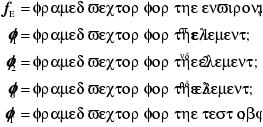The environment is the context within which the joint is moving. For the upper cervical spine, it may be the third cervical vertebra or even the axis. In many analyses, the environment will be the universal coordinate system for the body. For instance, anterior, lateral, and superior frame of reference vectors and the location of the axis vertebral body in space.

The test object is the object that we are monitoring for location and orientation. In the upper neck system, it might be the skull. Often it is the element of the system that we are studying as a reference for the entire system.

There are a number of possible mathematical structures that one might superimpose on the upper cervical joint assembly. Which one is used would depend on what information is available and what information is sought from the model. If we are primarily interested in the moving elements, then we might modify the standard framed vector by adding a second extension vector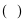, which is aligned with the axis of rotation. The location vector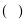would point to the center of the element, the first extension vector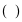might extend from the center of the element to the axis of rotation, and the second extension vector would be the axis of rotation. The frame of reference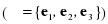would be the frame for the element.

We will generally not be reconstructing the objects, but it should be possible, in principle, to do so, if necessary. For instance, one might describe a ring centered upon the center and in the plane of the first and second elements of the orientation frame of reference as follows.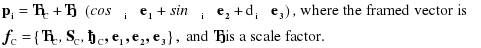## Methods

The calculations described in the Results Section were programmed in Mathematica Version 5. A collection of special functions were written to define framed vectors, frames of reference, and the operations that can performed upon them using quaternion analysis. The basic quaternion functions are in the standard add-on package Algebra `Quaternions`. The framework of the program is as described for the sample program in the Results.

## The Basic Format of the Analysis

Studies of the upper neck indicate that there are three orthogonal axes of movement for the atlanto-occipital joint that come near to intersecting in a common point (Kapandji 1974; Williams, Bannister et al. 1995; Levangie and Norkin 2001). The discrepancies are small relative to the dimensions of the head and thorax so it is reasonable to assume a common intersection for the purposes of a first analysis. Where questions depend on a very precise definition of the axes of rotation, it is relatively easy to go back into the model, make the changes, and re-run the calculations. The advantage of assuming a common intersection for all of the axes of the atlanto-occipital joint is that it simplifies many of the equations and the logic of the analysis

Most of the axes of rotation are not known with any greater precision than that used in the model. Perhaps the observations arising from these calculations will stimulate measurements that are more precise. On the other hand, the available precision is about a good as it gets for this type of measurement in a group of individuals. When examining bones taken from a variety of individuals, it is clear that there is considerable variation from spine to spine, therefore there may be little benefit in trying to achieve great precision, since it is apt to be empty precision.

For each structural element in the AAOA, we create a framed vector that has a standard set of components. The first component is the locus of the element (), which we are taking to be the center of the element. The second component is a center of rotation for the element (). The center of rotation is a point upon the axis of rotation. If there is more than one direction of rotation, then there may be more than one center of rotation. For instance, if flexion occurs about one axis and lateral rotation occurs about another that may not intersect that for flexion. We will assume a common intersection of the three orthogonal axes of rotation of the occiput upon the atlas, therefore there will be a common center of rotation for all three axes of rotation. The third component of the framed vector is a vector parallel with the axis of rotation (), that is, it is the direction of the axis of rotation. They are arranged so that the first is the offset of the axis of rotation, that is the chosen center of rotation, and the second is the axis of rotation. The last component f the framed vector is an array of three vectors that codes the orientation of the element (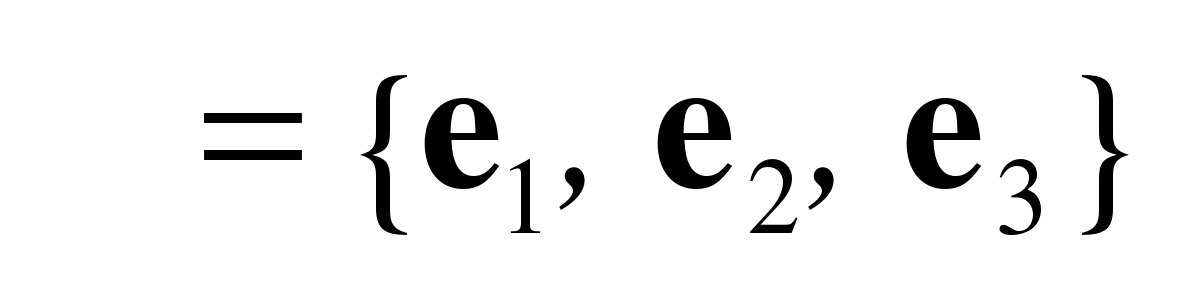). The set of axes used to code orientation is arbitrary, but it is usually convenient to choose them so that one can relate the anatomy of the structure to the orientation. For instance, the orientation of the skull is most logically related to the alignment of the eyes and/or the semicircular canals, since those are structures that the nervous system probably monitors for alignment.

These six vectors form the standard framed vector for the following analysis, but it may be convenient to extract certain of the components for parts of the analysis or to double up some of the elements where the structural element moves in different ways at different times. We will examine the consequences of rotations in the AAOA, by examining the results of manipulating these framed vectors that abstract the individual elements.